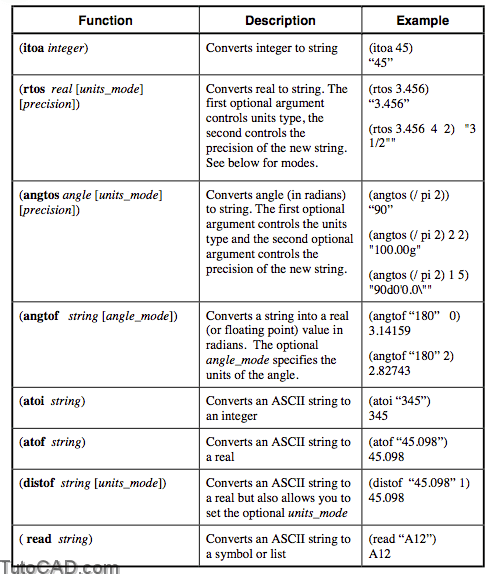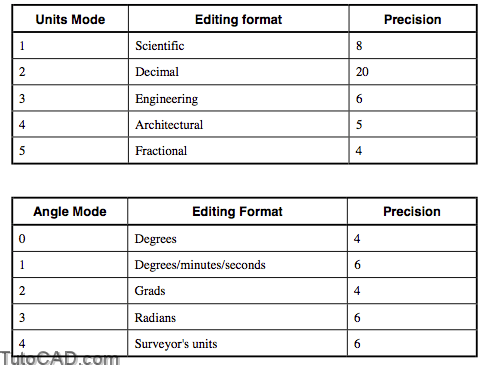# Converting Data Types with autolisp in AutoCAD

Since the (prompt) function must be a string, you will frequently need to convert information in a different data type to a string. We have done this repeatedly with the (itoa) function from an integer to a string. There are also functions that convert reals and angles to strings. Of course you may also need to change strings back to integers, reals, or angles.

Explanation• Remember, real numbers are calculated and stored using at least 14 significant digits, although only 6 are displayed to the user in AutoLISP. They must be converted to strings to be displayed longer.
• The “f” in several functions stands for “floating point,” another name for real numbers.
• The function (float) changes an integer to a real and the function (fix) truncates rather than rounds a real to an integer.

Units & Angle Modes

The functions (angtos), (rtos) and (distof) can be displayed using various unit and angle settings. For example, you may want the angles to display in degrees, minutes and seconds or the distance to be in architectural units.• If no mode or precision argument is supplied, the current settings of AUNITS and AUPREC are used for (angtos) and LUNITS and LUPREC for (rtos) and LUNITS for (distof).

Translating Unit Types: (cvunit)

If you are working with English and Metric units you may also need a function that converts a value from one unit of measurement to another.

(cvunit amount from_unit to_unit)

The amount is the integer or real you want to convert. The from_unit is the existing type of unit and the to_unit is the new unit type.

• The types of units you can choose from are found in the ACAD.UNT file.
• If you try to translate between two incompatible units (such as “gallons” and “years”) the function will return nil.
• If you are converting many numbers in a program you may want to convert the number 1.0 once. Then you can use the resulting number as a scale factor.

(setq SCALE_FACTOR(cvunit 1.0 “in” “mm”))

Examples

Command: (cvunit 2.00 “in” “mm”)
50.8

Command: (cvunit 2 “in” “m”)
0.0508

(defun C:IN2MM ( / INCHES MM BEFORE AFTER)
(initget (+ 1 2))
(setq INCHES (getdist “\nEnter a distance in inches:
“))
(setq MM (cvunit INCHES “inches” “millimeters”))
(setq BEFORE (rtos INCHES 2 4)
AFTER (rtos MM 2 4))
(princ
(strcat “\n\t” BEFORE ” inches = ” AFTER “millimeters.”))
(princ)
)

• A complete solution to this example is on your class disk as IN2MM.LSP

PRACTICE

Create a command line function, STAMP, that will allow the user to place a 1⁄4” high text string at a point in their drawing that contains the current date and time formatted such as 12/16/1999 11:46. Use the CDATE system variable to get the current date and time expressed as a real number. Try doing it on your own, only look at the solution when you get stuck. Estimated time for completion: 15 minutes.

Solution

(defun c:STAMP ()
(setq PT (getpoint “\nTime stamp insertion point: “)
CD-R (getvar “cdate”)
CD-S (rtos CD-R 2 4)
YR (substr CD-S 1 4)
MO (substr CD-S 5 2)
DY (substr CD-S 7 2)
HR (substr CD-S 10 2)
MN (substr CD-S 12 2)
DT(strcatMO“/”DY“/”YR“ ”HR“:”MN)
)
(entmake (list (cons 0 “TEXT”)
(cons 1 DT)
(cons 10 PT)
(cons 40 0.25)
)
)
(princ)
)

• A complete solution to this exercise is on your class disk as STAMP-A.LSP.